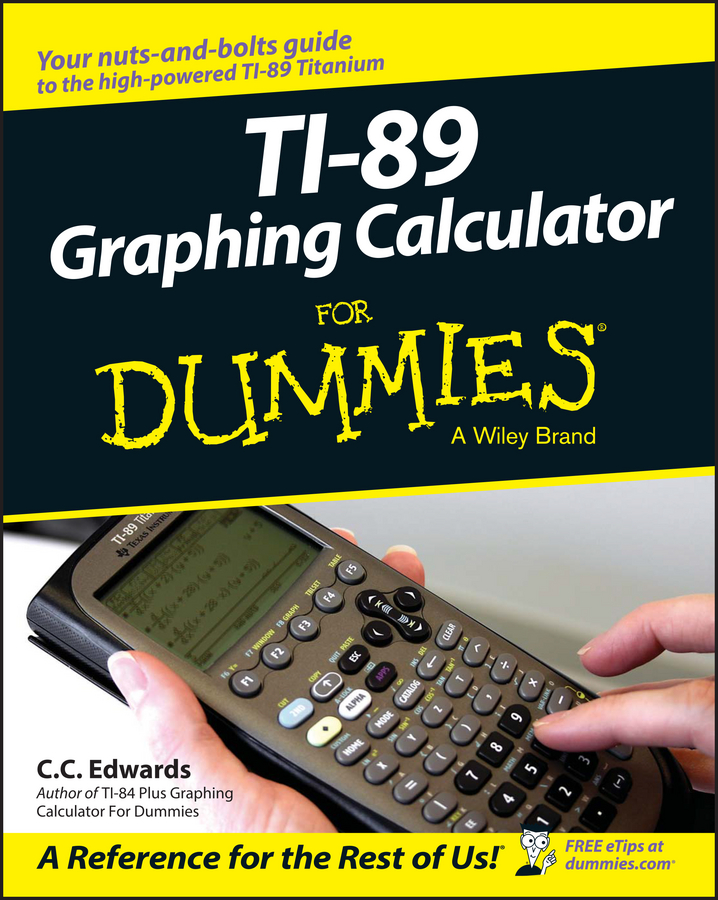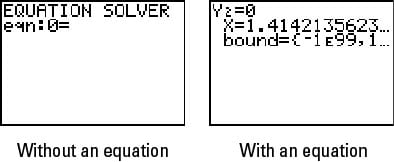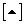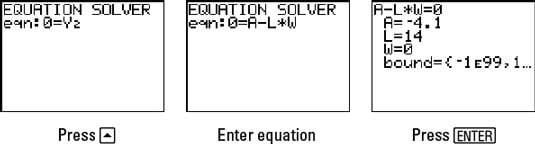##### TI-89 Graphing Calculator For DummiesThe second step of solving an equation in the TI-84 Plus graphing calculator's Equation Solver is to enter or edit the equation to be solved. The first step is to set the mode. To enter a new equation in the Equation Solver, follow these steps:

1. Press [MATH] to access the Equation Solver from the Math menu.The Equation Solver appears. The first screen shows the Equation Solver when no equation is stored in the Solver; the second picture depicts the Solver and the equation currently stored in it.The screen titled EQUATION SOLVER appears.3. If you need to, press [CLEAR] to erase any equation in the Solver and enter the equation you want to solve.

The equation you enter must be set equal to zero and can contain only real numbers. For example, if you want to solve the area equation A = L*W, enter it as 0 = A – L*W or as 0 = L*W – A.

4. Press [ENTER] to enter the equation in the solver.

You also can edit an equation that is already entered in the Equation Solver by repeating the first two steps and pressing [ENTER] when done.